# Abstract Algebra : Geometric Fields

## Example Questions

### Example Question #1 : Abstract Algebra

Identify the following definition.

For some subfield of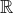, in the Euclidean plane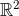, the set of all points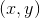that belong to that said subfield is called the __________.

Constructible Line

Angle

Plane

Line

Plane

Explanation:

By definition, whenis a subfield of, in the Euclidean plane, the set of all pointsthat belong tois called the plane of.

### Example Question #10 : Abstract Algebra

Identify the following definition.

Given thatlives in the Euclidean plane. Elements, andin the subfieldthat form a straight line who's equation form is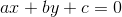, is known as a__________.

Line inSubfield

Plane

Angle

Circle inLine inExplanation:

By definition, given thatlives in the Euclidean plane. When elements, andin the subfield, form a straight line who's equation form is, is known as a line in.

### Example Question #1 : Fields

Identify the following definition.

Given thatlives in the Euclidean plane. Elements, andin the subfieldthat form a straight line who's equation form is, is known as a__________.

Angle

Plane

Circle inSubfield

Line inLine inExplanation:

By definition, given thatlives in the Euclidean plane. When elements, andin the subfield, form a straight line who's equation form is, is known as a line in.

### Example Question #2 : Geometric Fields

Identify the following definition.

If a line segment has length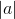and is constructed using a straightedge and compass, then the real numberis a __________.

Angle

Constructible Number

Plane

Magnitude

Straight Line

By definition if a line segment has lengthand it is constructed using a straightedge and compass then the real numberis a known as a constructible number.